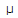# Electronics - Magnetism and Electromagnetism - Discussion

### Discussion :: Magnetism and Electromagnetism - General Questions (Q.No.3)

3.

What is the flux density of a magnetic field whose flux is 3000 µWb and cross-sectional area is 0.25 m2?

 [A]. 12,000T [B]. 83,330 T [C]. 0 T [D]. More information is needed in order to find flux density.

Explanation:

No answer description available for this question.

 Meena said: (Mar 5, 2011) B=u*H H=B/u H=3000/0.25 H=12000uT

 Sonal said: (Sep 3, 2011) fi=B*A B=fi/A B=3000/.25 B=12000

 Alok Kumar Mishra said: (Oct 18, 2013) Given: Area = 0.25m^2. flux = 3000*10^-6. flux = magnetic flux density*area. mfd = flux/area. mfd = 3000*10^-6/.25. mfd = 12000 ut.

 Shreya said: (Dec 16, 2013) So simple flux density means flux per unit area.

 Pooh... said: (Mar 5, 2014) What is the flux?

 Nemai said: (Mar 20, 2014) Flux density = Wb/m2.

 Mohammad Saad said: (Apr 9, 2014) Simple flux density means flux per unit area. mfd = flux/area.

 Ankita Anmol said: (Oct 19, 2014) Mag.flux density = flux/unit area.

 Rex K B Andoh said: (Nov 25, 2014) Magnetic flux density, B = Magnetic flux,(PHI)/Area, A. = 3000*10^-6/0.25. = 12000 UT.

 Manoj said: (Jan 30, 2015) MAGNETIC FLUX DENSITY = MAGNETIC FLUX/AREA. B = 3000*10^-6/0.25 = 12000 MICRO WEBER/M^2.

 Kainat.. said: (Feb 4, 2015) The flux passing through unit area gives flux density.

 Samir Ali said: (Oct 25, 2016) Two type's of flux density. They are: 1) Magnetic flux density. 2) Electric flux density. For magnetic flux density: B(flux density) = Q(flux)/A(area). For electric flux density: D(flux density) = c/area.

 Anurag Mishra said: (Aug 5, 2017) Q= B/A, = 3000/0.25. = 12000 micro-tesla.

 Diptikanta Sahu said: (Feb 19, 2018) Here, D=B/A, =3000/0.25.

 Abdihalim Abdinor said: (Jan 21, 2019) Solution: Flux = magnetic field/cross-sectional area. = 3000/.25. =12000μT.